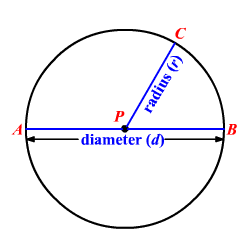# Diameter

In a plane, a circle is the set of all points equidistant from a given point called the center. You can name a circle by its center. Circle $P\left(\odot P\right)$ is shown.A radius is a segment that has one endpoint at the center and the other endpoint on the circle. In the circle $P$ , $PC$ is the radius. $PA$ and $PB$ are also radii.

A diameter is a segment that contains the center of a circle and has both endpoints on the circle. In the circle $P$ , $AB$ is a diameter.